Courses

# Test: Geometrical Isomerism- 1

## 22 Questions MCQ Test Chemistry for JEE | Test: Geometrical Isomerism- 1

Description
This mock test of Test: Geometrical Isomerism- 1 for JEE helps you for every JEE entrance exam. This contains 22 Multiple Choice Questions for JEE Test: Geometrical Isomerism- 1 (mcq) to study with solutions a complete question bank. The solved questions answers in this Test: Geometrical Isomerism- 1 quiz give you a good mix of easy questions and tough questions. JEE students definitely take this Test: Geometrical Isomerism- 1 exercise for a better result in the exam. You can find other Test: Geometrical Isomerism- 1 extra questions, long questions & short questions for JEE on EduRev as well by searching above.
QUESTION: 1

### Direction (Q. Nos. 1-13) This section contains 13 multiple choice questions. Each question has four  choices (a), (b), (c) and (d), out of which ONLY ONE option is correct. Q. Which among the following defines Meso forms of isomers

Solution:

a) False, meso form is optically inactive due to presence of Plane of symmetry, centre of symmetry or axis of symmetry and not due to external compensations.
b) False, since meso is optically inactive, so they are achiral
c) False, they can’t be separated into optically active enantiomeric pairs
d) True, it is a single compound.

QUESTION: 2

### The molecular formula C5H12 contains how many isomeric alkanes?

Solution:

To have three isomeric monochlorides, the isomer of the alkane of the molecular formula C5H12 should contain three different types of H-atoms. Therefore, the isomer is n-pentane. It can be observed that there are three types of H atoms labelled as a, b and c in n-pentane.QUESTION: 3

### The molecular formula C5H12 contains how many isomeric alkanes?

Solution:

n-pentane, 2-ethylpropane, and 2-methylbutane are the 3 isomeric alkanes of C5H12 (pentane).

QUESTION: 4

How many alcohols are structural isomers with the formula: C5H11OH?

Solution:

Structural isomers of alcohol C5H11OH are as follow:-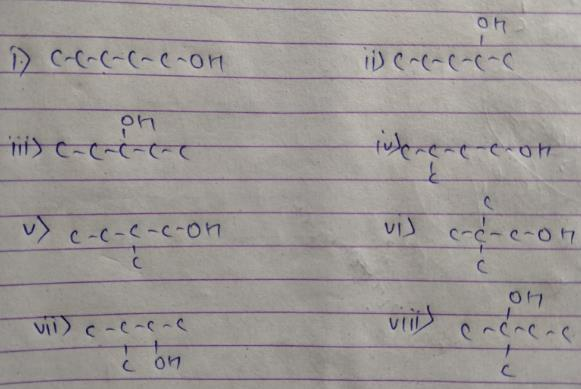QUESTION: 5

A cyclic dichloride has a total five constitutional plus geometrical isomers. Which of the following satisfy this condition without altering the carbon skeleton?

Solution: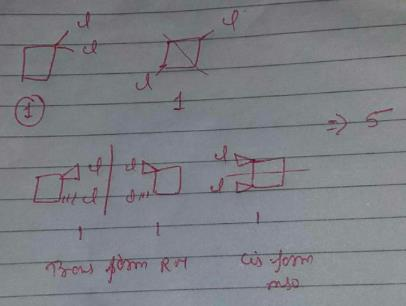Only option b exists with 5 constitutional + geometrical isomers without altering carbon skeleton.

QUESTION: 6

Which of the following carbonyls has four different enol isomers?

Solution: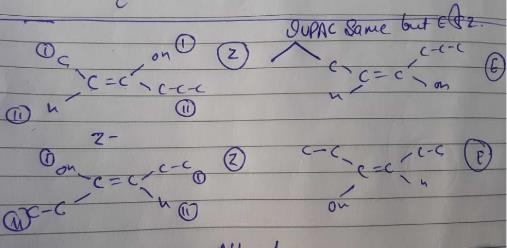The given picture starts with an enol form of 3-hexanone.

QUESTION: 7

How many different stereoisomers exist for 1-chloro-2-(3-chlorocyclobutyl) ethene?

Solution: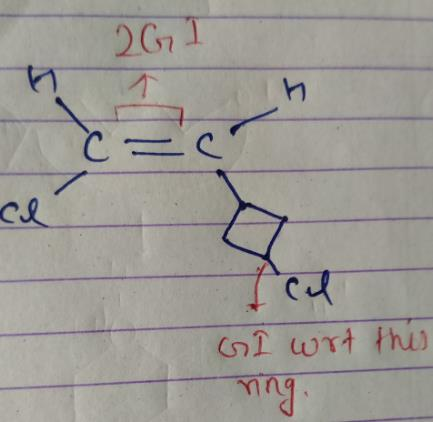So, there are 4 isomers of given compound.

QUESTION: 8

Which of the following has three different stereoisomers?

Solution:

The correct answer is Option B.

CH3−CH=CH−CH=CH−CH3

Three different geometrical isomers of cis-cis, trans-trans and sic-trans are possible.

QUESTION: 9

Which among the following does not exhibit geometric isomerism

Solution:

Alkenes like 1-hexene when flipped from top to bottom they have identical structures and also they have C=CH2 unit which does not exist as cis- trans isomers.

QUESTION: 10

'Which carbonyls below, on protonation gives a pair of stereoisomers?

Solution:

The correct answer is option C

In chemistry, protonation (or hydronation) is the addition of a proton (or hydron, or hydrogen cation), (H+) to an atom, molecule, or ion, forming the conjugate acid. Some examples include. the protonation of water by sulfuric acid:
H2SO4 + H2O ⇌ H3O+ + HSO

QUESTION: 11

A stereoisomer of cyclobutane-1,2-diol has lower solubility in water than its other stereoisomer. Which is this isomer and why?

Solution:
QUESTION: 12

How many cyclic isomers exists (structural and geometrical only) for C3H3CI3

Solution: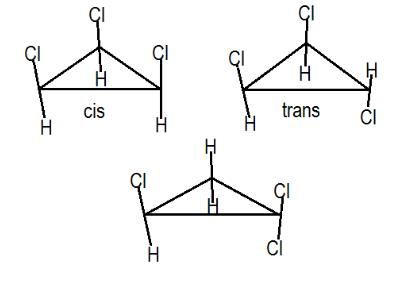These 3 are the possible structures.
Hence, B is correct.

QUESTION: 13

which compound below can show geometrical isomerism ?

Solution:

(a)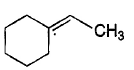We have the same arrangement if the -CHand -H are interchanged. So, no GI.
(b)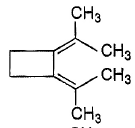Also in this compound we will get same arrangement across double bond if the positions of both -CH3 are interchanged around  a single bond
(c)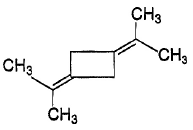Same case here as in the above two.
(d)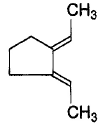Here, if we interchange -CH3 and -H around a double bond, then we will get a different arrangement with respect to other double bond. So it shows GI.

*Multiple options can be correct
QUESTION: 14

Direction (Q. Nos. 14-18) This section contains 5 multiple choice questions. Each question has four
choices (a), (b), (c) and (d), out of which ONE or  MORE THANT ONE  is correct.

Q.

Which compound below can have a non-polar steroisomers ?

Solution: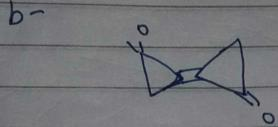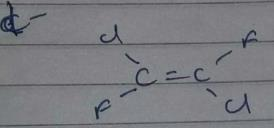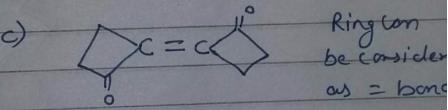However, there is no such arrangement in structure i, it won’t have any non polar structure.

*Multiple options can be correct
QUESTION: 15

Which of the following is/are expected to form significant intramolecular H-bond?

Solution:

According to the definition of intramolecular H bonding, a H atom attached with F, O and N is attracted by highly electronegative atoms (N, O, and F) within the molecule. So, by following the definition, only c and d are expected to form intramolecular H bonding.

*Multiple options can be correct
QUESTION: 16

Choose the correct statement(s) regarding geometrical isomerism?

Solution:

Statement a) true, GI have the same constitutions. Only the position of groups is changed.
Statement b) False, Even without pi bond we have GI. eg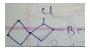Statement c) True, Smaller cycloalkenes have only cis configuration at double bonds to maintain the planarity of bond
Statement d) True, cyclodecene exists with both cis and trans configuration at double bond.

*Multiple options can be correct
QUESTION: 17

Which of the following compounds will show geometrical isomerism?

[IIT JEE 1998]

Solution:

The correct answer is Option A and C.

CH3 - CH = H - CH3 shows the property of geometrical isomerism.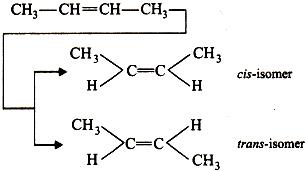CH3 - CH = CH2 does not show the property of geometrical isomerism.

CH3 - CH = CH - C6H5 shows the property of geometrical isomerism.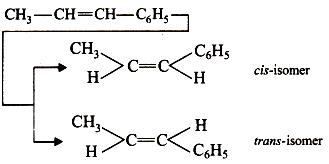(CH3)2 - C = CH - CH3 does not show the property of geometrical isomerism.

*Multiple options can be correct
QUESTION: 18

The correct statements(s) concerning the structures E,F and G is (are)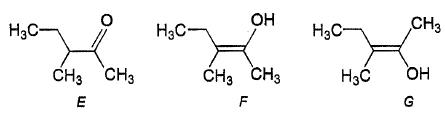[IIT JEE 2008]

Solution:

The correct answers are option B,C,D
The correct statements concerning the  structures E, F and G are:
(B) E, F and  G are tautomers.
E is in keto form (C=O) and F and G are in enol form (C=C−OH)
(C) F and G are geometrical isomers.
F is Z isomer and E is E isomer.
(D) F and G are diastereomers. They are not mirror images of each other and non-superimposable.

QUESTION: 19

Direction (Q. Nos. 19-22) This sectionis based on statement I and Statement II. Select the correct answer from the code given below

Q.

Statement I Fluoroethanal has two stereomeric enols in which cis enol predominates at equilibrium.

Statement II Intramolecular H-bonding increases the stability of a stereomer.

Solution:
QUESTION: 20

Q.

Statement I : Butanone, upon protonation at oxygen atom result in a pair of stereoisomer.

Statement II : Presence of lone pair of electrons at oxygen is responsible for protonation.

Solution:
QUESTION: 21

Q.

Statement I :  1,2-dichlorocyclopropane has two stereoisomers.
Statement II : Restricted rotation is responsible for cis and trans-isomers of 1,2-dichlorocyclopropane.

Solution:

In order to show stereoisomers, a compound should not contain plain of symmetry,centre of symmetry,or any
In cis- form of this compound plain of symmetry will be present making the molecule incapable to show sterioisomerism
So statement 2 is correct, while statement 1  is incorrect.

QUESTION: 22

Q.

Statement I : 1,2,3,4-tetrachloro-1,3-butadiene has two non-polar stereoisomers,

Statement II : Out of its four stereoisomers, two of them are polar.

Solution: